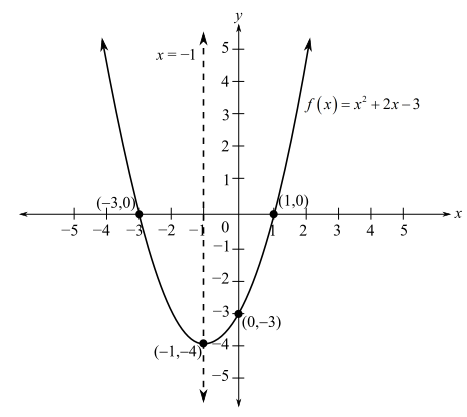Chapter 2, Problem 1REFinite Mathematics and Applied Cal...

7th Edition
Stefan Waner + 1 other
ISBN: 9781337274203

Solutions

Chapter
SectionFinite Mathematics and Applied Cal...

7th Edition
Stefan Waner + 1 other
ISBN: 9781337274203
Textbook Problem

Sketch the graph of the quadratic functions in Exercises 1 and 2, indicating the coordinates of the vertex, the y-intercept, and the x-intercepts (if any). f ( x ) = x 2 + 2 x − 3

To determine

To graph: The function f(x)=x2+2x3 and indicate the coordinates of the vertex, the y-intercept, and x-intercept.

Explanation

Given Information:

The provided function is f(x)=x2+2x3.

Graph:

Consider the function,

f(x)=x2+2x3

Compare the equation f(x)=x2+2x3 with the standard function f(x)=ax2+bx+c and find the value of a,b and c.

The values are a=1,b=2 and c=3.

Here a>0, therefore the parabola will be upward facing,

To graph a quadratic function, four things should be calculated first.

(i) Vertex

(ii) x-intercept

(iii)y-intercept

(iv) Symmetry

Vertex: The formula of x- coordinate of a vertex is,

x=b2a

Substitute a=1 and b=2 in the equation x=b2a.

x=221=22=1

To find the y-coordinate of vertex, substitute x=1 in the function f(x)=x2+2x3.

f(x)=(1)2+3(1)+2=13+2=0

Thus, coordinates of the vertex are (1,0).

To calculate the x-intercept of the function, substitute f(x)=0 in the equation f(x)=x2+2x3 and solve.

x2+2x3=0x2+3xx3=0x(x+3)1(x+3)=0(x+3)(x1)=0

Therefore, x+3=0 or x1=0.

Now solve for x+3=0.

x+3=0x=3

Now solve for x1=0.

x1=0x=1

Thus, x-intercept are 3, 1.

To calculate the y-intercept, substitute x=0 in the function f(x)=x2+2x3.

f(x)=(0)2+2(0)3=3

Thus, the y-intercept is 3.

Symmetry:

The formula of the symmetry line is,

x=b2a

Substitute a=1 and b=2 in the equation x=b2a.

x=221=22=1

So, the line of symmetry is x=1.

Now plot the graph.Interpretation:

The graph of f(x)=x2+2x3 represents a parabola which is upward facing. Also, the vertex is (1,4), the y-intercept 3, and x-intercept are 3 and 1.

The Solution to Your Study Problems

Bartleby provides explanations to thousands of textbook problems written by our experts, many with advanced degrees!

Get Started

In Exercises 1-6, simplify the expression. 3+2x7x

Calculus: An Applied Approach (MindTap Course List)

In Exercises 99-106, factor out the greatest common factor from each expression. 106. 12(23u3/22u1/2)

Applied Calculus for the Managerial, Life, and Social Sciences: A Brief Approach

In problems 13-16, Use notation to indicate which set is a subset of the other. 15.

Mathematical Applications for the Management, Life, and Social Sciences

Factor completely. 2x2+x1

Trigonometry (MindTap Course List)

It does not exist.

Study Guide for Stewart's Multivariable Calculus, 8th

In the graph at the right, limx1f(x)=. a) 2 b) 1 c) 3 d) does not exist

Study Guide for Stewart's Single Variable Calculus: Early Transcendentals, 8th

Find all the answers to your study problems with bartleby.
Textbook solutions plus Q&A. Get As ASAP arrow_forward